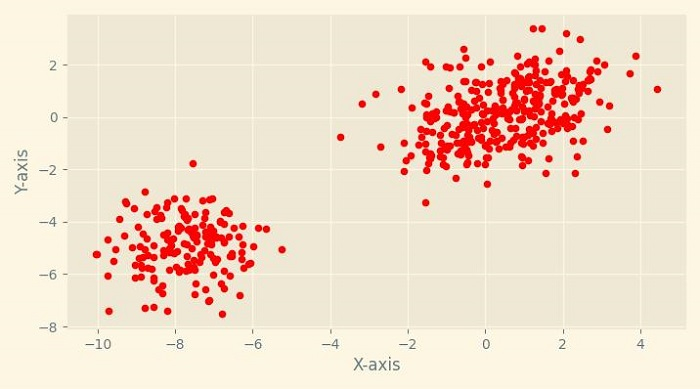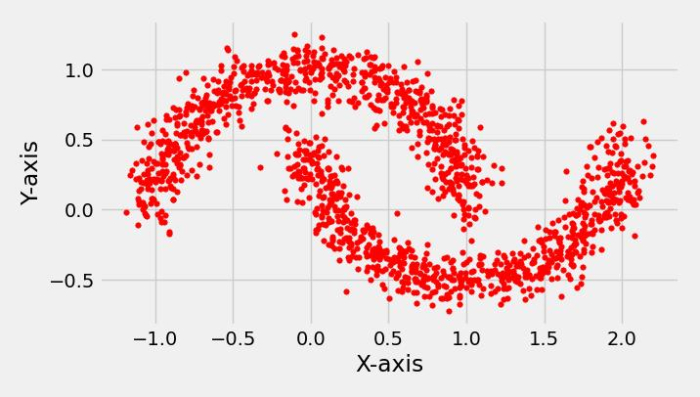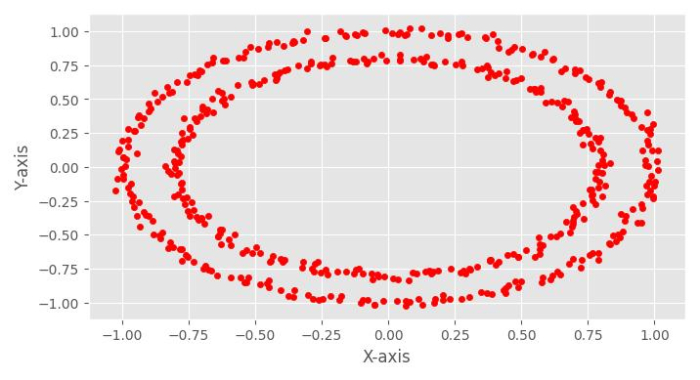# How to create a sample dataset using Python Scikit-learn?

In this tutorial, we will learn how to create a sample dataset using Python Scikit-learn.

There are various built-in scikit-learn datasets which we can use easily for our ML model but sometimes we need some toy dataset. For this purpose, scikit-learn python library provides us a great sample dataset generator.

## Creating Sample Blob Dataset using Scikit-Learn

For creating sample blob dataset, we need to import sklearn.datsets.make_blobs which is very fast and easy to use.

### Example

In the below given example, let’s see how we can use this library to create sample blob dataset.

# Importing libraries
from sklearn.datasets import make_blobs

# Matplotlib for plotting the dataset blobs
from matplotlib import pyplot as plt
from matplotlib import style

# Set the figure size
plt.rcParams["figure.figsize"] = [7.50, 3.50]
plt.rcParams["figure.autolayout"] = True

# Creating Blob Test Datasets using sklearn.datasets.make_blobs

style.use("Solarize_Light2")

X, y = make_blobs(n_samples = 500, centers = 3,
cluster_std = 1, n_features = 2)

plt.scatter(X[:, 0], X[:, 1], s = 20, color = 'red')
plt.xlabel("X-axis")
plt.ylabel("Y-axis")
plt.show()


### Output

It will produce the following output −The above output shows it created 3 blobs from 500 samples.

## Creating Sample Moon Dataset using Scikit-Learn

For creating sample moon dataset, we need to import sklearn.datsets.male_moons which is very fast and easy to use.

### Example

In the below given example, let’s see how we can use this library to create sample moon dataset.

# Importing libraries
from sklearn.datasets import make_moons

# Matplotlib for plotting the moon dataset
from matplotlib import pyplot as plt
from matplotlib import style

# Set the figure size
plt.rcParams["figure.figsize"] = [7.16, 3.50]
plt.rcParams["figure.autolayout"] = True

# Creating Moon Test Datasets using sklearn.datasets.make_moon
style.use("fivethirtyeight")
X, y = make_moons(n_samples = 1500, noise = 0.1)
plt.scatter(X[:, 0], X[:, 1], s = 15, color ='red')
plt.xlabel("X-axis")
plt.ylabel("Y-axis")
plt.show()


### Output

It will produce the following output## Creating Sample Circle Dataset using Scikit-Learn

For creating sample circle dataset, we need to import sklearn.datsets.make_circles which is very fast and easy to use.

### Example

In the below given example, let’s see how we can use this library to create sample circle dataset.

# Importing libraries
from sklearn.datasets import make_circles

# Matplotlib for plotting the circle dataset
from matplotlib import pyplot as plt
from matplotlib import style

# Set the figure size
plt.rcParams["figure.figsize"] = [7.16, 3.50]
plt.rcParams["figure.autolayout"] = True

# Creating the circle Test Datasets using sklearn.datasets.make_circles
style.use("ggplot")
X, y = make_circles(n_samples = 500, noise = 0.02)
plt.scatter(X[:, 0], X[:, 1], s = 20, color ='red')
plt.xlabel("X-axis")
plt.ylabel("Y-axis")
plt.show()


### Output

It will produce the following output −